International
Tables for
Crystallography
Volume D
Physical properties of crystals
Edited by A. Authier

International Tables for Crystallography (2006). Vol. D, ch. 1.1, p. 5

## Section 1.1.2.1. Change of basis

A. Authiera*

aInstitut de Minéralogie et de la Physique des Milieux Condensés, Bâtiment 7, 140 rue de Lourmel, 75015 Paris, France
Correspondence e-mail: aauthier@wanadoo.fr

#### 1.1.2.1. Change of basis

| top | pdf |

Let us consider a vector space spanned by the set of n basis vectors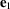,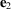,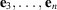. The decomposition of a vector using this basis is written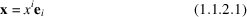using the Einstein convention. The interpretation of the position of the indices is given below. For the present, we shall use the simple rules:

 (i) the index is a subscript when attached to basis vectors; (ii) the index is a superscript when attached to the components. The components are numerical coordinates and are therefore dimensionless numbers.

Let us now consider a second basis,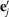. The vector x is independent of the choice of basis and it can be decomposed also in the second basis: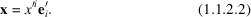If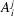and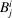are the transformation matrices between the basesand, the following relations hold between the two bases: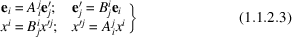(summations over j and i, respectively). The matricesandare inverse matrices: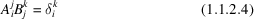(Kronecker symbol: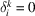if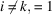if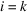).

Important Remark. The behaviour of the basis vectors and of the components of the vectors in a transformation are different. The roles of the matricesandare opposite in each case. The components are said to be contravariant. Everything that transforms like a basis vector is covariant and is characterized by an inferior index. Everything that transforms like a component is contravariant and is characterized by a superior index. The property describing the way a mathematical body transforms under a change of basis is called variance.